SLVSAC5 April   2014

PRODUCTION DATA.

1. Features
2. Applications
3. Description
4. Revision History
5. Device Comparison Table
6. Terminal Configuration and Functions
7. Specifications
8. Parameter Measurement Information
9. Detailed Description
1. 9.1 Overview
2. 9.2 Functional Block Diagram
3. 9.3 Feature Description
4. 9.4 Device Functional Modes
10. 10Applications and Implementation
1. 10.1 Application Information
2. 10.2 Typical Application
11. 11Power Supply Recommendations
12. 12Layout
13. 13Device and Documentation Support
14. 14Mechanical, Packaging, and Orderable Information

• YFF|6

## 7 Specifications

### 7.1 Absolute Maximum Ratings

over operating free-air temperature range (unless otherwise noted)(1)
MIN MAX UNIT
VI Voltage at VIN(2) –0.3 6 V
Voltage at FB(2) –0.3 3.6
Voltage at SW, EN, AVIN (2) –0.3 VIN + 0.3
Continuous average output current(4) 890 mA
Peak output current(4) 1600 mA
TJ Operating junction temperature(3) -40 150 °C
(1) Stresses beyond those listed under absolute maximum ratings may cause permanent damage to the device. These are stress ratings only and functional operation of the device at these or any other conditions beyond those indicated under recommended operating conditions is not implied. Exposure to absolute-maximum-rated conditions for extended periods may affect device reliability.
(2) All voltage values are with respect to network ground terminal.
(3) In applications where high power dissipation and/or poor package thermal resistance is present, the maximum ambient temperature may have to be derated. Maximum ambient temperature (TA(max)) is dependent on the maximum operating junction temperature (TJ(max)), the maximum power dissipation of the device in the application (PD(max)), and the junction-to-ambient thermal resistance of the part/package in the application (θJA), as given by the following equation: TA(max)= TJ(max)–(θJA X PD(max)). To achieve full lifetime, it is recommended to operate the device with a maximum junction temperature of 105°C.
(4) Limit the junction temperature to 105°C.

### 7.2 Handling Ratings

MIN MAX UNIT
Tstg Storage temperature range –65 150 °C
ESD rating (1) Human body model 2 kV
Charge device model 1
Machine model 100 V
(1) The human body model is a 100-pF capacitor discharged through a 1.5-kΩ resistor into each pin. The machine model is a 200-pF capacitor discharged directly into each pin.

### 7.3 Recommended Operating Conditions

MIN NOM MAX UNIT
VIN Input voltage range 3.25 5.5 V
IO Peak output current(1) VIN < VOUT,nom + 1V 0 960 mA
VOUT,nom + 1V ≤ VIN ≤ 5.5V 0 1600
CI Effective Input Capacitance(2)(3) 0.5 µF
L Effective Inductance 0.3 1.2 µH
CO Effective Output Capacitance(2) 3.0 5.0 30 µF
TA Ambient temperature(4) –40 +85 °C
TJ Operating junction temperature(5) –40 +125 °C
(1) Operating beyond the continuous average output current of 890mA may decrease the lifetime. See the Thermal, Lifetime Information and Maximum Output Current section.
(2) Due to the dc bias effect of ceramic capacitors, the effective capacitance is lower than the nominal value when a voltage is applied. The capacitance is specified to allow the selection of the appropriate capacitor taking into account its dc bias effect.
(3) Larger values may be required if the source impedance can not support the transient requirements of the load.
(4) In applications where high power dissipation and/or poor package thermal resistance is present, the maximum ambient temperature may have to be derated. Maximum ambient temperature (TA(max)) is dependent on the maximum operating junction temperature (TJ(max)), the maximum power dissipation of the device in the application (PD(max)), and the junction-to-ambient thermal resistance of the part/package in the application (θJA), as given by the following equation: TA(max)= TJ(max)–(θJA X PD(max)). To achieve full lifetime, it is recommended to operate the device with a maximum junction temperature of 105°C.
(5) Limit the junction temperature to 105°C at 1.6A output current for a lifetime of 25k hours.

### 7.4 Thermal Information

THERMAL METRIC(1) TPS62684 UNIT
YFF
6 TERMINALS
RθJA Junction-to-ambient thermal resistance 108.9 °C/W
RθJC(top) Junction-to-case (top) thermal resistance 1.0
RθJB Junction-to-board thermal resistance 17.5
ψJT Junction-to-top characterization parameter 4.1
ψJB Junction-to-board characterization parameter 17.5
RθJC(bot) Junction-to-case (bottom) thermal resistance n/a

### 7.5 Electrical Characteristics

Minimum and maximum values are at VI = 3.25V to 5.5V, EN = VIN and TA = –40°C to 85°C; Circuit of Parameter Measurement Information section (unless otherwise noted). Typical values are at VIN = 3.6V, EN = VIN and TA = 25°C (unless otherwise noted).
PARAMETER TEST CONDITIONS MIN TYP MAX UNIT
SUPPLY CURRENT into VIN + AVIN
IQ Operating quiescent current IO = 0mA 5.8 mA
ISD Shutdown current EN = low; not including high side MOSFET leakage
0.2 1.5 μA
VUVLO Undervoltage lockout threshold VIN rising 2.1 2.3 V
VIN falling 1.95 2.1 V
ENABLE
VIH High-level input voltage 0.9 V
VIL Low-level input voltage 0.4 V
Ilkg,EN Input leakage current EN connected to GND or VIN; TJ = –40°C to 85°C 0.01 0.1 μA
POWER SWITCH
RDS(on),HS High Side MOSFET on resistance VIN = 3.6V; TJ = –40°C to 125°C 95 155
VIN = 2.5V 170
Ilkg,HS High Side MOSFET leakage current VIN = 5.5V; TJ = –40°C to 85°C 2.6 μA
RDS(on),LS Low Side MOSFET on resistance VIN = 3.6V; TJ = –40°C to 125°C 75 155
VIN = 2.5V 100
Ilkg,LS Low Side MOSFET leakage current VIN = 5.5V; TJ = –40°C to 85°C 1 μA
Resistor in parallel to Low Side MOSFET 250
RDIS Discharge resistor for power-down sequence only active after a first power-up (EN = high to low after VIN applied) 12 Ω
Average High Side MOSFET current limit 1680 2100 2850 mA
Input current limit under short-circuit conditions VOUT shorted to ground 150 mA
Thermal shutdown Temperature rising 140 °C
Thermal shutdown hysteresis Temperature falling 10 °C
OSCILLATOR
fSW Nominal oscillator frequency IOUT = 0mA 5.5 MHz
OUTPUT
VOUT,nom Nominal output voltage 2.85 V
Output voltage accuracy 3.25V ≤ VIN ≤ 3.85V, 0mA ≤ IO ≤ 960 mA 0.98×VOUT,NOM VOUT,NOM 1.02×VOUT,NOM V
3.85V ≤ VIN ≤ 5.5V, 0mA ≤ IO ≤ 1600 mA 0.98×VOUT,NOM VOUT,NOM 1.02×VOUT,NOM V
Line regulation VIN = VOUT + 0.5V (min 3.25V) to 5.5V, IO = 200 mA 0.2 %/V
Load regulation IO = 0mA to 1600 mA –0.00085 %/mA
FB pin input resistance 1.4

### 7.6 Timing Requirements

MIN TYP MAX UNIT
Start-up delay time IO = 0mA, Time from EN = high to start switching 120 300 µs
tRAMP ramp time IO = 0mA, Time from start switching until 95% of nominal output voltage 150 µs
Shutdown time IO = 0mA, Time from EN = low to VO < 500mV, Effective Output Capacitance CO_effective = 5µF 300 µs

### 7.7 Typical Characteristics

TABLE OF GRAPHS FIGURE
η Efficiency vs Load current Figure 1, Figure 2, Figure 3, Figure 4
vs Input voltage Figure 5
Load transient response Figure 8, Figure 9, Figure 10, Figure 11, Figure 12, Figure 13, Figure 14
AC load transient response Figure 15
Line Transient Response Figure 16
VOUT DC output voltage vs Load current Figure 6, Figure 7
fsw PWM switching frequency vs Input voltage Figure 17
PWM switching frequency vs Load Current Figure 18
PWM operation Figure 19
Spread spectrum frequency modulation operation Figure 20
Start-up Figure 21, Figure 22
Shutdown Figure 23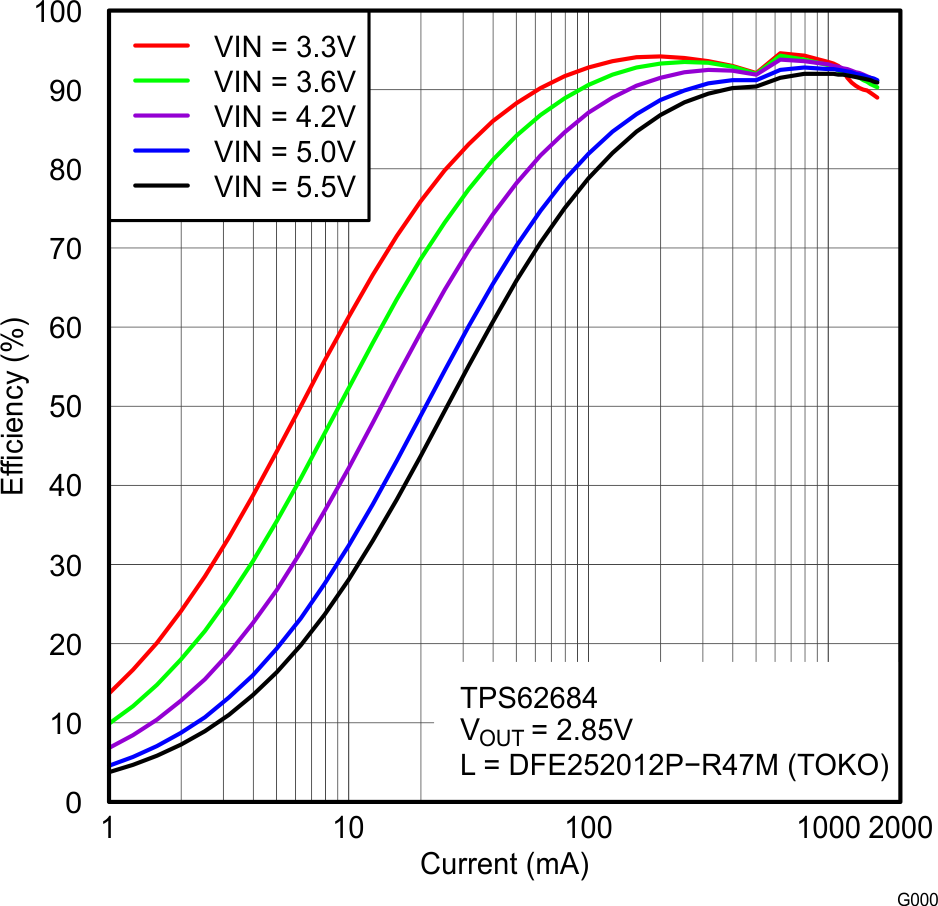Figure 1. Efficiency Vs Load Current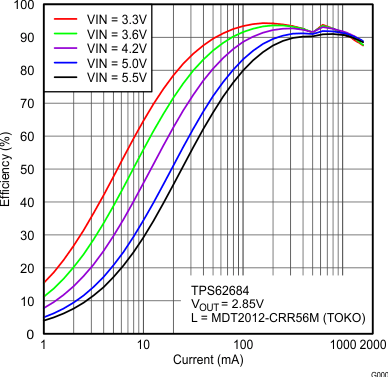Figure 2. Efficiency Vs Load Current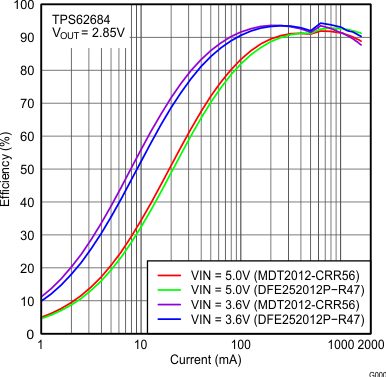Figure 3. Efficiency Vs Load Current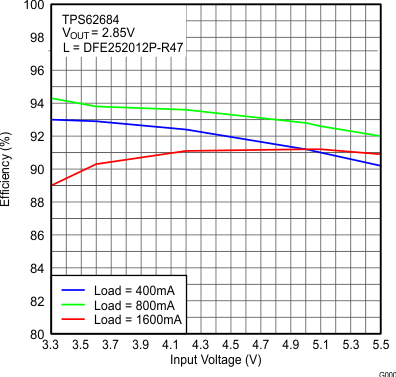Figure 5. Efficiency Vs Input Voltage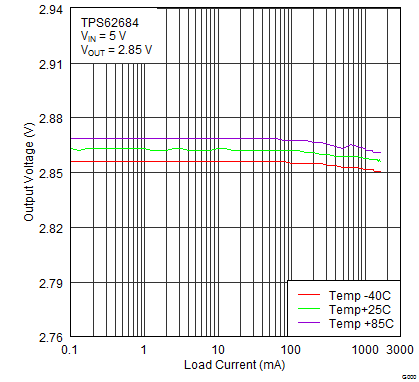Figure 7. Output Voltage Vs Load Current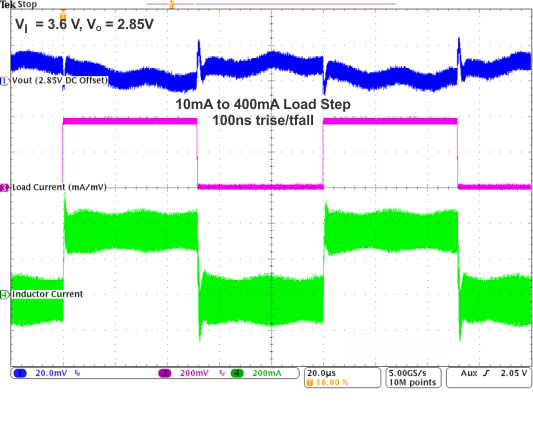Figure 9. Load Transient Response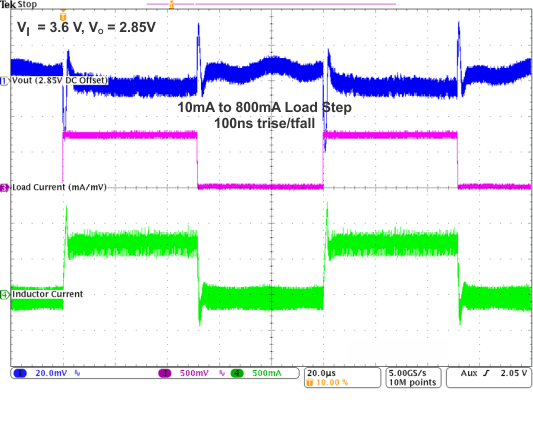Figure 11. Load Transient Response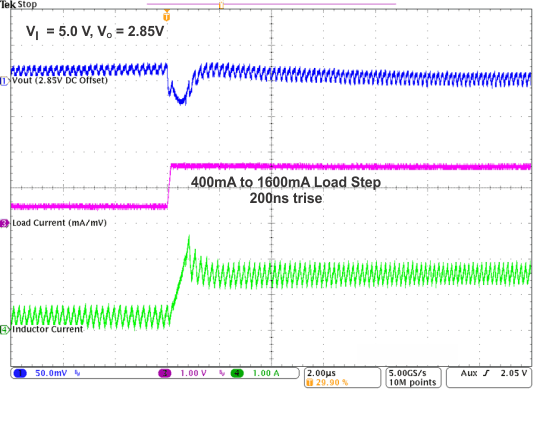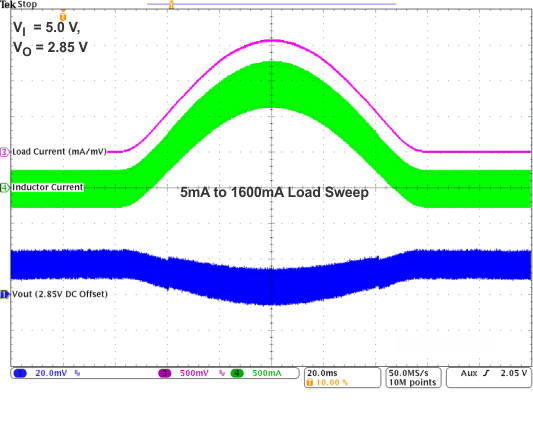Figure 15. AC Load Transient Response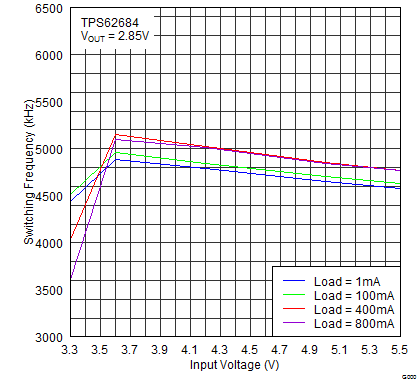Figure 17. PWM Switching Frequency Vs Input Voltage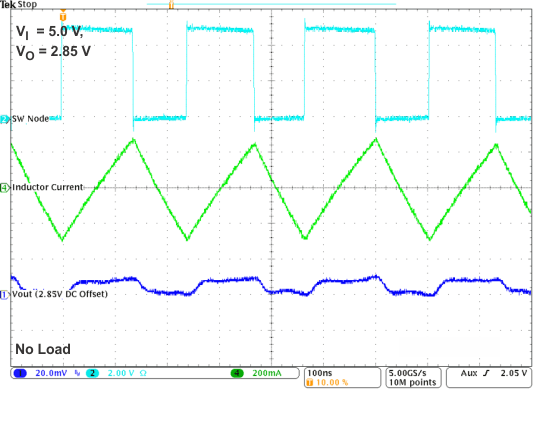Figure 19. PWM OperationFigure 21. Start-Up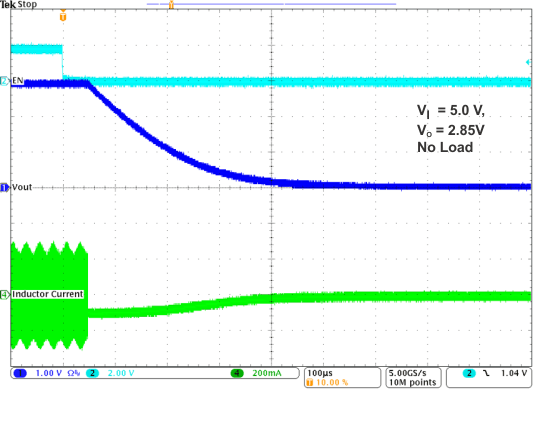Figure 23. Shutdown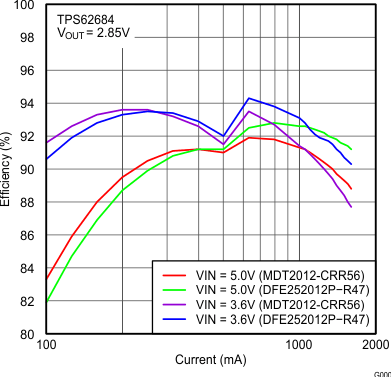Figure 4. Efficiency Vs Load Current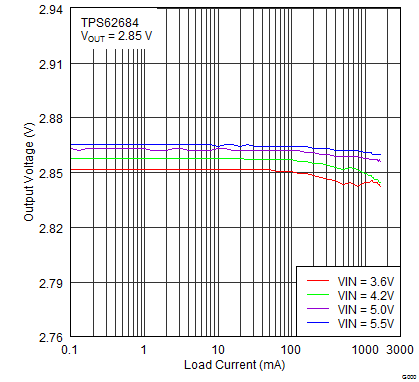Figure 6. Output Voltage Vs Load Current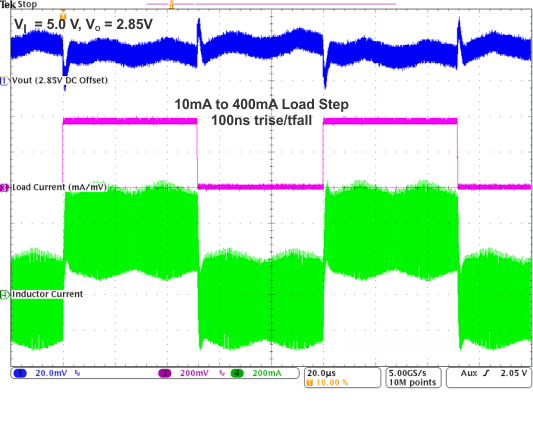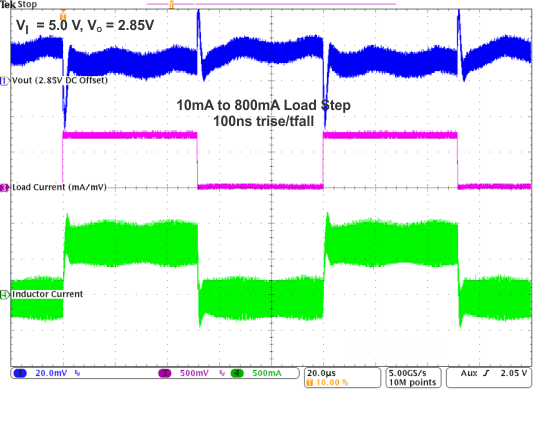Figure 10. Load Transient Response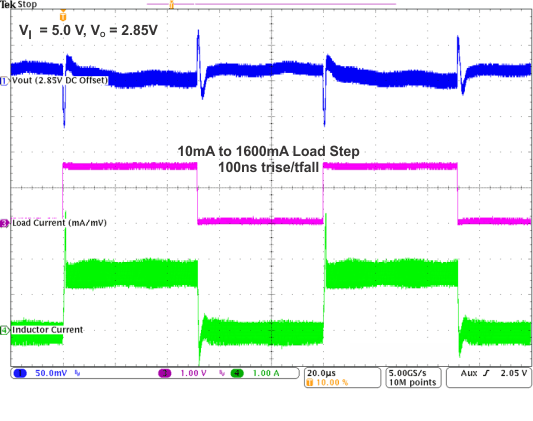Figure 12. Load Transient Response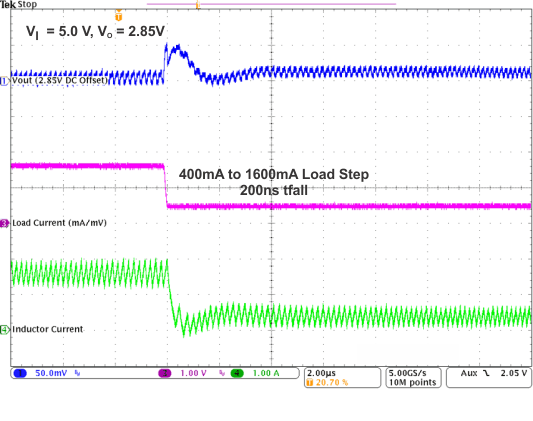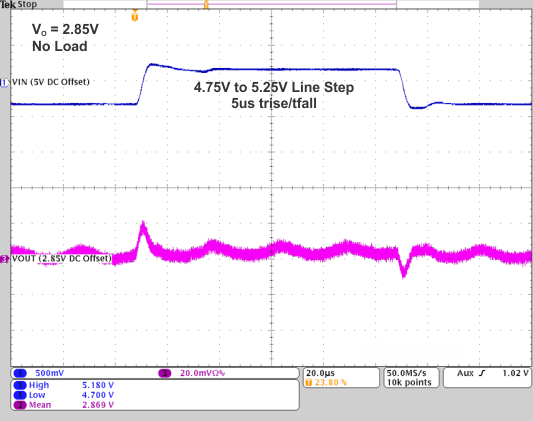Figure 16. Line Transient Response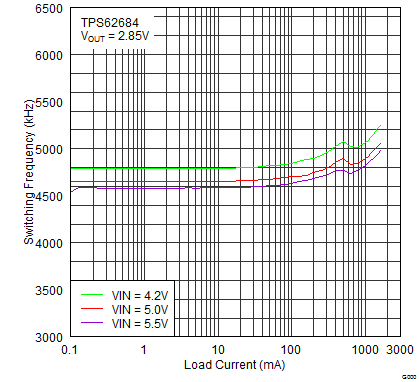Figure 18. PWM Switching Frequency Vs Load Current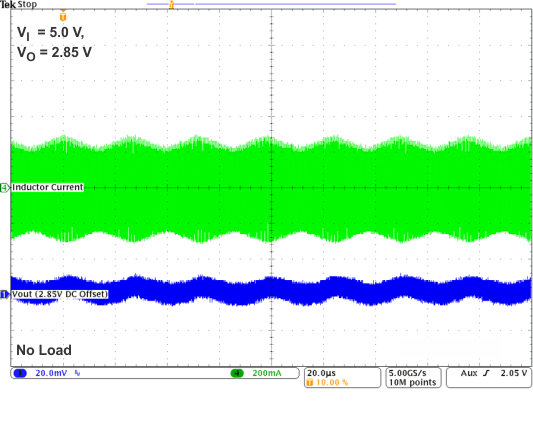Figure 20. Spread Spectrum Frequency Modulation Operation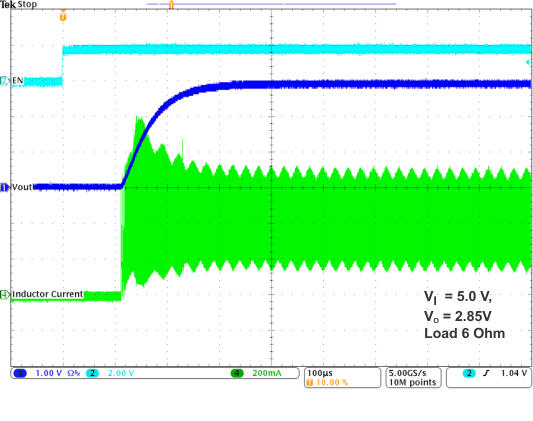Figure 22. Start-Up